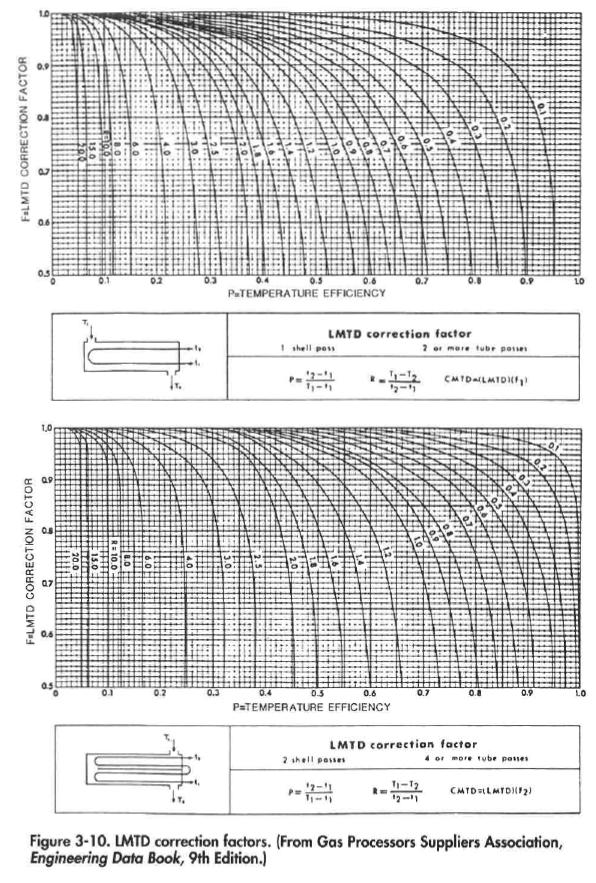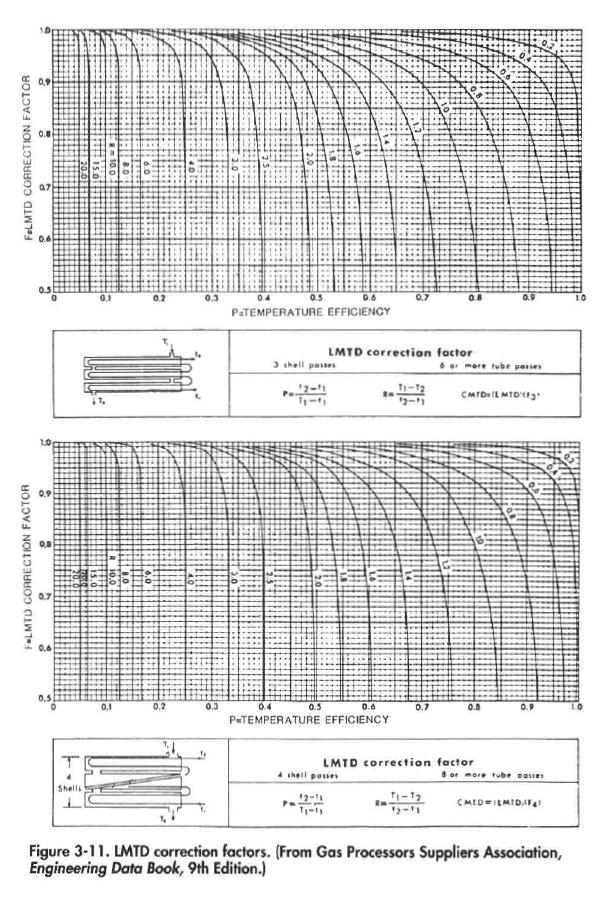﻿ Shell and Tube Heat Exchanger Sizing | Oil & Gas Process Engineering

## Shell and Tube Heat Exchanger Sizing

The required heat duty, film coefficients, conductivity, etc. for a shelland-tube heat exchanger can be calculated using the procedures in Heat Transfer Theory, Approximate U-values are given in Table 2-8.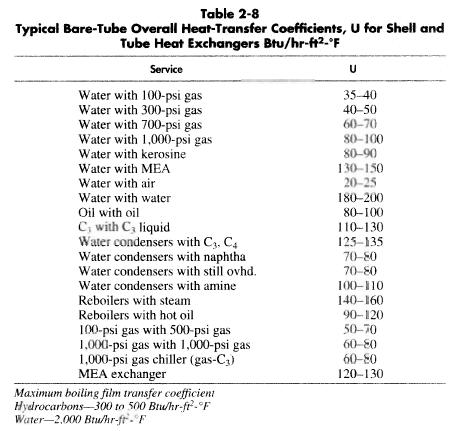In the basic heat transfer equation it is necessary to use the log mean temperature difference. In Equation 2-4 it was assumed that the two fluids are flowing counter-current to each other. Depending upon the configuration of the exchanger, this may not be true. That is, the way in which the fluid flows through the exchanger affects LMTD. The correction factor is a function of the number of tube passes and the number of shell passes.

Figures 3-10 and 3-11 can be used to calculate a corrected LMTD from the formula.To size a shell-and-tube exchanger, first the duty is calculated. Then it is determined which fluid will be in the shell and which in the tube, and a heat* transfer coefficient assumed or calculated. A choice is made of the number of shell and tube passes to get a reasonable LMTD correction factor (F), and a corrected LMTD as calculated from Equation 3-1.

Next, a tube diameter and tube length are chosen. The number of tubes required is calculated by: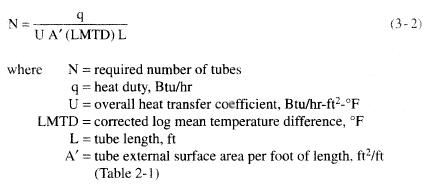From Table 3-4 it is then possible to pick a shell diameter that can accommodate the number of tubes required. Please note that Equation 3-2 calculates the total number of tubes required and not the number of tubes per pass. Similarly, Table 3-4 lists the total number of tubes and not the number per pass. There are fewer total tubes in the same diameter exchanger for more passes of the tube fluid because of the need for partition plates. There are fewer tubes for floating head than fixed head
designs because the heads and seals restrict the use of space. U-tubes have the lowest number of tubes because of the space required for the tightest radius bend in the U-tube bundle.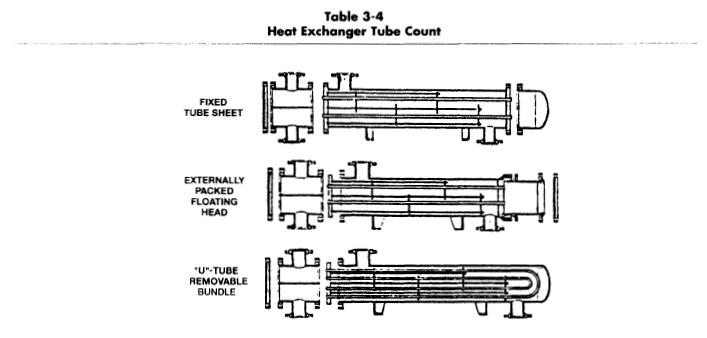Once the number of tubes is determined, the flow velocity of fluid inside the tubes should be checked, using the criteria set forth for flow in pipes.